# Difference between BQM, Ising, and QUBO problems?

I have seen the mathematical expressions for Ising and QUBO. Other than the fact that Ising deals with spin and QUBO works with binary, the expressions look identical to me. Is there a significant difference between the two problems? Also, how does BQM fit into this picture?

Ising problem: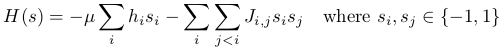QUBO problem: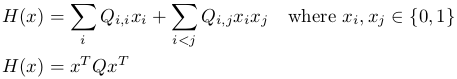• BQM stands for Binary Quadratic Model and is just a general term that encompasses both Ising and QUBO problems.
• The Ocean software (libraries for running problems on D-Wave quantum computers) accepts either Ising or QUBO problems, but flags may need to be turned on to indicate whether we are interested in a "BINARY" (QUBO) or "SPIN" (Ising) solution.
• The expressions for an Ising and for a QUBO problem do look very similar. In fact, the Ising and QUBO expressions are isomorphic. (See "Proof that Ising and QUBO are isomorphic")
• Find out more on BQMs: binary-quadratic-model-doc
• For an understanding of what Ising and QUBOs are: problem-formulations-ising-and-qubo

### Extension to differences between Ising and QUBO

Yes, the major difference between Ising  and QUBO is that Ising deals with spin (-1, 1), while QUBO uses binary (0,1).  While the two expressions are isomorphic, the choice of spin or binary can effect the way the problem can be expressed; namely, why QUBOs can always be fully expressed in both expanded and matrix forms, while Ising can be fully expressed in the expanded form, but not completely in the matrix form. Consider the following matrix multiplication,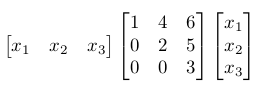(Eq. 1)

If we expanded this matrix multiplication, we get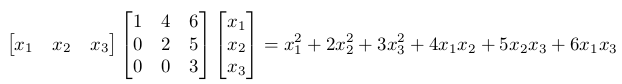(Eq. 2)

Now recall that we can express both Ising and QUBO problems in the following expanded form: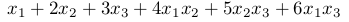(Eq. 3)

Recall with QUBOs, we are only dealing with binary values, 0s and 1s. Therefore if we have an x_i from the set {0,1}, then x_i is always equal to x_i^2. (i.e. 0=0^2 and 1=1^2). This means that a QUBO problem expressed as Eq. 3 (shown on the left hand side), can also be expressed as Eq. 2 (shown on the right hand side).However, this property of x_i = x_i^2 is not true for spins. As in -1 does not equal (-1)^2. Therefore, the Ising problem can not be written completely in matrix form.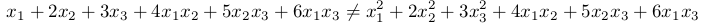### Third Term Expansion

We want the third term in terms of x_i. Let's expand and see what we can do.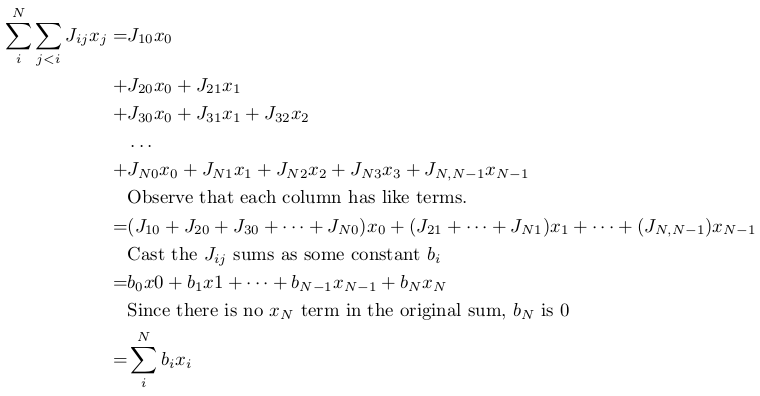• Hello Melody Wong, thank you for taking time explaining above. What would be the benefit of using Ising, if not all problems can be represented using it, but using Qubo, you can do it? why bother then thinking Ising direction at all?

• Hello Branislav,

Good question! QUBOs are easily expressed in Matrix form, as Melody explained above. However, both QUBO and Ising formats can be useful for different kinds of problems.

Take the first two Leap demos, for example. In the Factoring demo, it is useful to write numbers in standard binary notation (e.g. 101 = 5). Once our numbers are in this format, we can build a QUBO that multiplies these numbers, because the qubits in a QUBO can be represented as 0 or 1.

On the other hand, in the Social Network Analysis demo, we want to split people into two groups. We can say that a person (qubit) is on side A if they are +1, and side B if they are -1. It is useful that two people in the same group will always multiply to +1, because we can then "couple" two people together (make them tend to be in the same group) with J terms.

If you go through the Leap demos and click the "More Information" tabs, you can find more detail about how these examples make use of the Ising and QUBO formats.

• Hi Branislav,

Thank you for your interest! To add on to what Luca has said, there are several reasons why Ocean (D-Wave's open source software) supports Ising.

(1) Quantum processor uses Ising under the hood

• Recall that you are interacting with a quantum computer that is using sophisticated physics under the hood. This means that symmetry naturally occurs in all the equations used to calibrate and to set up the quantum computer.
• Example of symmetry: two magnetic fields of equal strength, say 5 Tesla. They are identical except that one magnetic field is pointed INTO the screen, while the other is pointed OUT of the screen. In this case, it is convenient to just use +/ - signs to indicate direction; namely +5 Tesla and -5 Tesla. These values can be used in further calculations where both the magnitude and direction are of interest.
• Since the quantum processor uses Ising, this means that QUBO equations get converted into Ising under the hood (Point A)

(2) Coefficient imbalance is more apparent in Ising

• Based on (Point A), when we use Ising, we can see exactly which coefficients are being fed to the QPU. Why is this useful?
• This is useful because the weights of an Ising problem work in a particular range. Namely, [-2,2] for linear weights and [-1, 1] for quadratic weights. When you exceed these weight ranges, the QPU will need to re-scale the problem to fit it into the mentioned ranges.
• So if you end up with an Ising equation like `1000*s0 + 0.5*s1 + 200*s0*s1`, you can already see that there may be issues in the future. Namely, the weight 0.5 will get re-scaled to something very small; this means that the constraint that the 0.5 was meant to represent might diminish. (Ideally, you want your coefficients to be around the same magnitude, so that re-scaling won't be a huge issue).

(3) Some concepts are more convenient to express in Ising rather than in QUBO

• As Luca has already mentioned, some concepts are more easily expressed in Ising than in QUBO, and vice versa
• An example where Ising is convenient. Consider the "Not Equals" relationship between a and b (i.e. the lowest energy states are when a does not equal b).

• Ising NOT-EQUALS: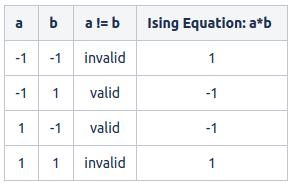• QUBO NOT-EQUALS: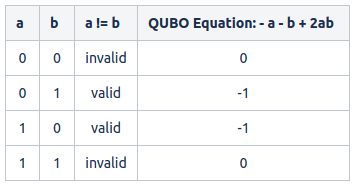• As seen in the equations above, while both Ising and QUBO can describe the Not Equals relationship, Ising is just more convenient in this case. Specifically, Ising can express the relationship in one simple term (a*b), while QUBO requires three terms (-a -b +2ab).